April 24, 2019

Convolutional Neural Networks

Convolutional neural networks present a different architecture that works a way better that common neural networks when we are dealing with images. As we have previously seen, a common neural network receives a 2 dimensional input, if we have n images of three dimensions (height x width x depth) we have to transform these 3 dimensions into one in order to obtain an input (n x the new dimension) of two dimensions. Therefore, if we have an image of (64 x 64 x 3) dimensions, we would need 12, 288 neurons in the input layer, those are a lot of neurons for such a small image. For instance if we want to use bigger images we must use a different architecture, in this post we will see how convolutional neural networks solve this problem.

Convolutional layer

This architecture uses two different layers instead of the dense layers common neural networks use. The first new layer is called convolutional layer, in this layer we use a parameter called kernel or filter to extract features from the image. Let’s see an example: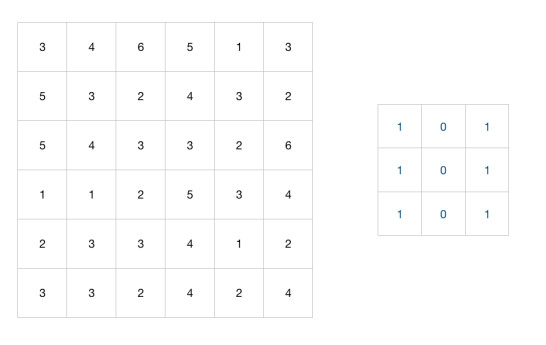In the image above we have an image represented as an array of size 6x6 and a kernel of size 3x3.

In order for the kernel to extract features from the image, it has to pass over the image and multiply each pixel of the image by the corresponding value of the kernel, this process is called the convolution operation: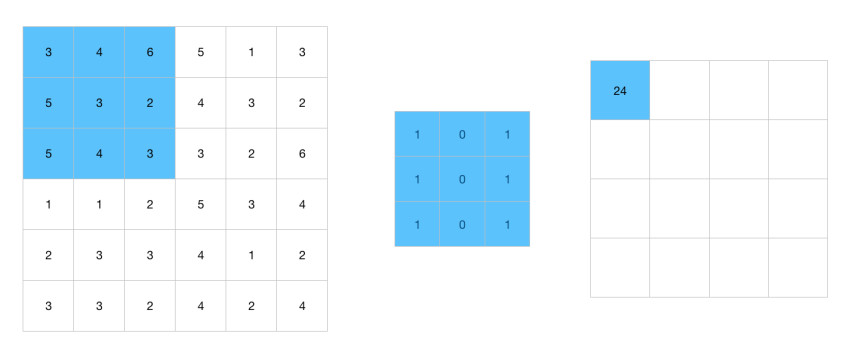First kernel column: 3 x 1 + 5 x 1 + 5 x 1 = 13

Second kernel column: 4 x 0 + 3 x 0 + 4 x 0 = 0

Third kernel column: 6 x 1 + 2 x 1 + 3 x 1 = 11

Finally we sum these results to obtain the first value of the output image:

13 + 0 + 11 = 24

The kernel will move over the image column by column until it reaches the last column: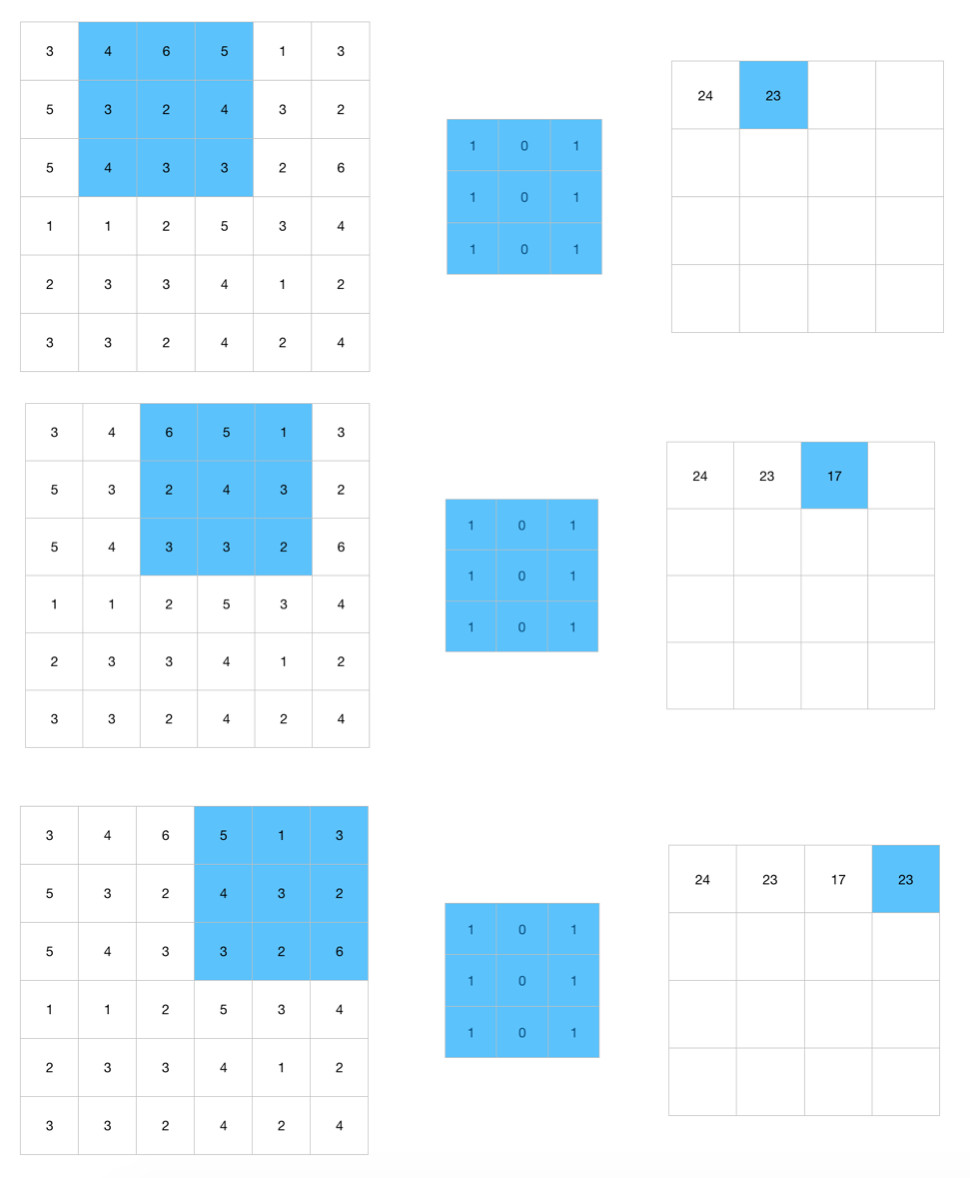Second value

4 x 1 + 3 x 1 + 4 x 1 = 11
6 x 0 + 2 x 0 + 3 x 0 = 0
5 x 1 + 4 x 1 + 3 x 1 = 12

11 + 0 + 12 = 23

Third value

6 x 1 + 2 x 1 + 3 x 1 = 11
5 x 0 + 4 x 0 + 3 x 0 = 0
1 x 1 + 3 x 1 + 2 x 1 = 6

11 + 0 + 6 = 17

Fourth value

5 x 1 + 4 x 1 + 3 x 1 = 12
1 x 0 + 3 x 0 + 2 x 0 = 0
3 x 1 + 2 x 1 + 6 x 1 = 11

12 + 0 + 11 = 23

The kernel will move over the image row by row and repeat the process: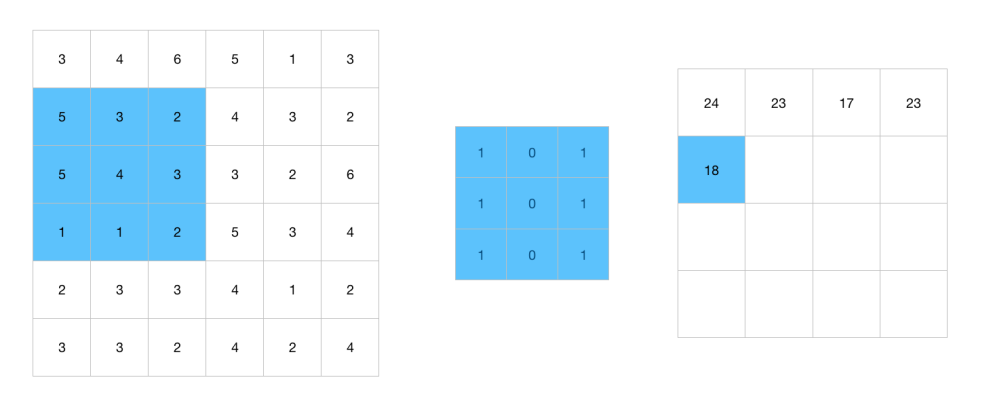We end up with the following output image: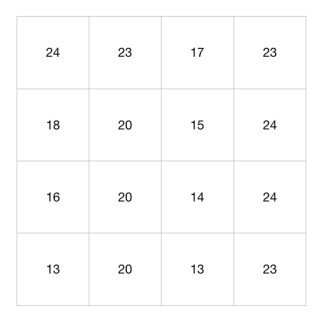In this layer we have the parameter b and we use an activation function to obtain an output as well: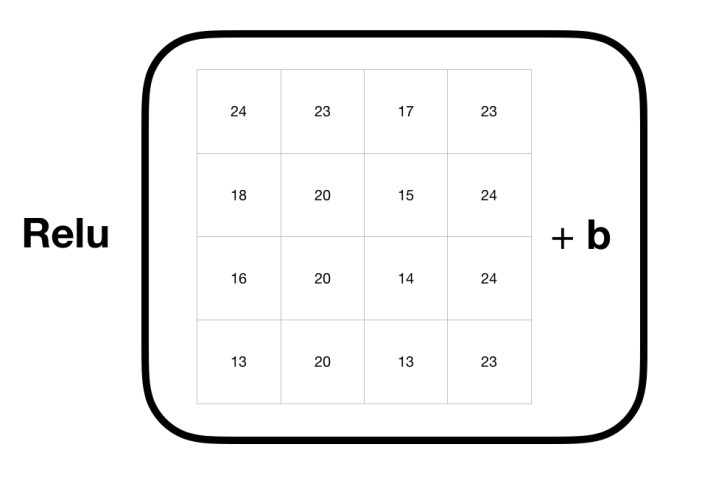You may realize that in this layer we don’t have a W parameter, in fact we could say that the kernel parameter substitutes the job of W.

We can use a method called Padding in the convolutional layers to add pixeles to the corners of the image: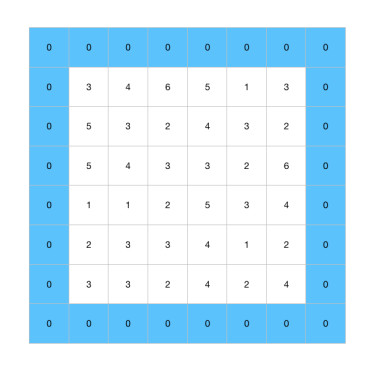Sometimes we have images that contain important information at the corners, suppose we have an image with people faces and some of these faces appear at the corners, if we compute the convolutional operation, the kernel will miss these faces due to the fact it only covers the corners of the image a few times, if we move these faces to the center of the image the kernel will be able to obtain more information from these faces.

We can also use this method to make the output image bigger, continuing with the example from the previous section we have an image of size 6x6 and a kernel of size 3x3, thus, the output image has a size of 4x4, in fact, in a convolutional neural network we have several convolutional layers, each time the image passes through a layer the output image will be smaller, we also have pooling layers, which we will see in the next section, that reduce the size of the output image furthermore, that’s why sometimes we need to add extra pixels to the image to avoid ending up with a really small output image.

Stride

This is a parameter used in the convolutional and pooling layers that indicates how many positions the kernel will move.

In the convolutional layer section we saw how the kernel moves through the image one column and one row at time but we can change this number.

We always start in the same position: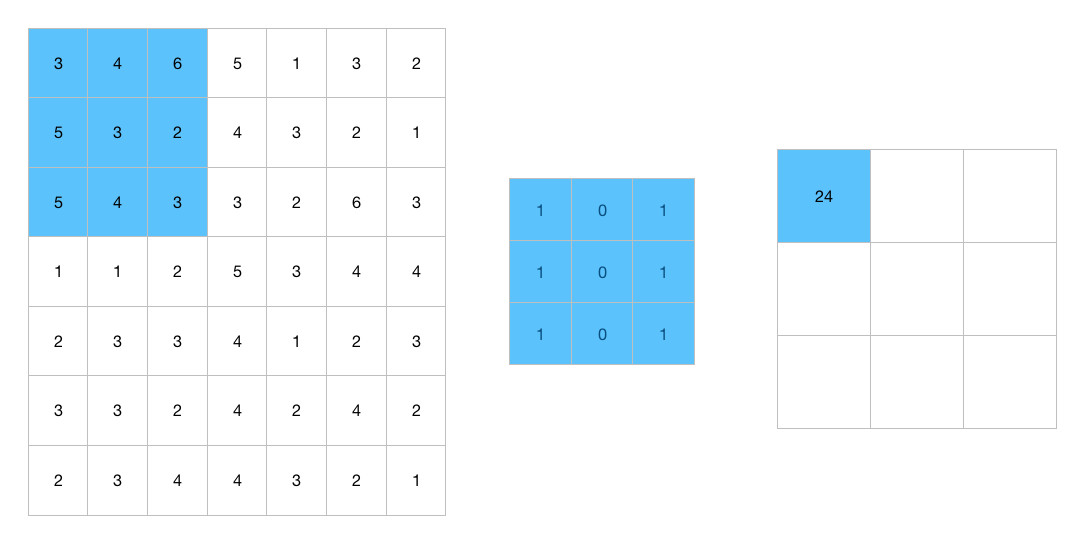But now the kernel will move two columns instead of one: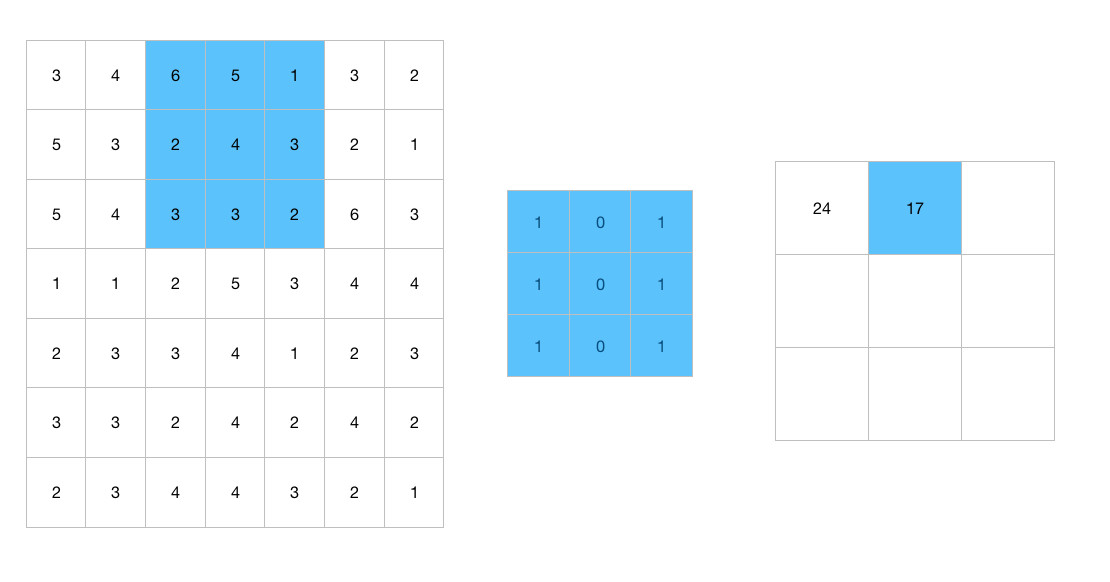It will move two rows as well: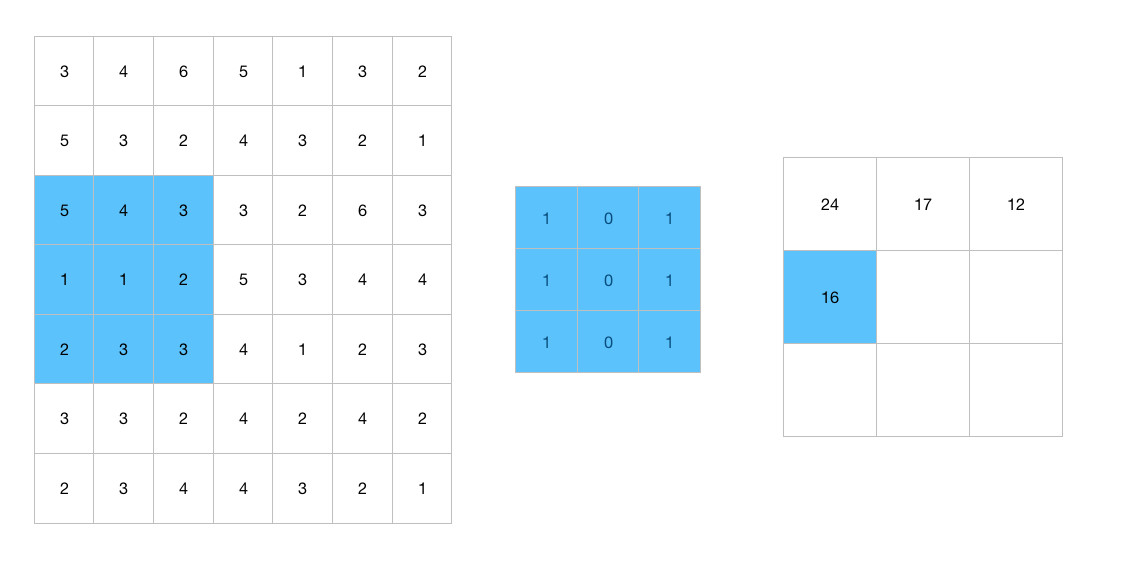If we use a bigger number the output image will be smaller and the convolution operation will be faster.

We must be carefully with the choose of this number, in the example above I used an image of size 7x7 instead of an image of size 6x6 in order to match the kernel 3x3 with the image, if I had used an image of size 6x6: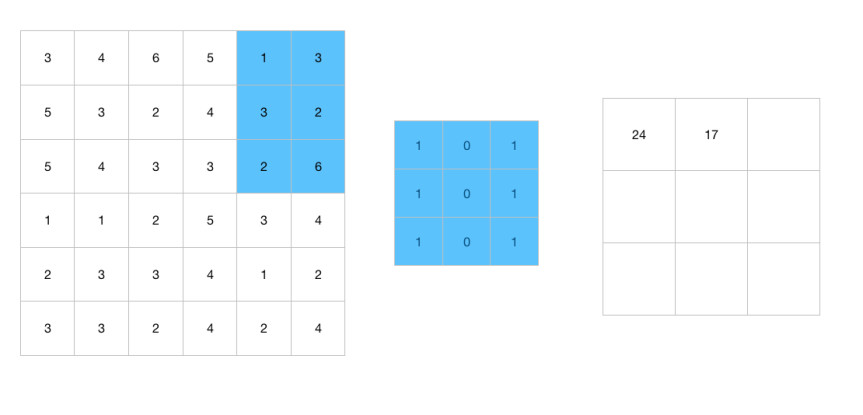The kernel would have not covered the image correctly

We can use a formula to solve this problem:

(N + 2p - k / s)  + 1 x (N + 2p - k / s)  + 1

This formula will tell us the size of the output image, N is the image’s size, K is the kernel’s size, p is the padding we used in the image and finally s is the number of positions the kernel will move (stride).

If we use an image of size 7x7 with 0 padding, a kernel of size 3x3 and a stride of 2:

(7 + 0 - 3 / 2)  + 1 x (7 + 0 - 3 / 2)  + 1
= (4 / 2) + 1 x (4 / 2) + 1
= 3 x 3

We obtain an output image of size 3x3, however if we use an image of size 6x6 with the same parameters:

(6 + 0 - 3 / 2)  + 1 x (6 + 0 - 3 / 2)  + 1
= (3 / 2) + 1 x (3 / 2) + 1
= 2.5 x 2.5

We obtain an output image of size 2.5x2.5, for instance we have to change some parameters in order to obtain a valid output image.

Color images

We have seen how convolutional layers works with grayscale images of depth one, but we often work with color images of depth 3 (RGB, 3 color channels), in this case the convolutional layer has 3 kernels, one kernel for each color channel: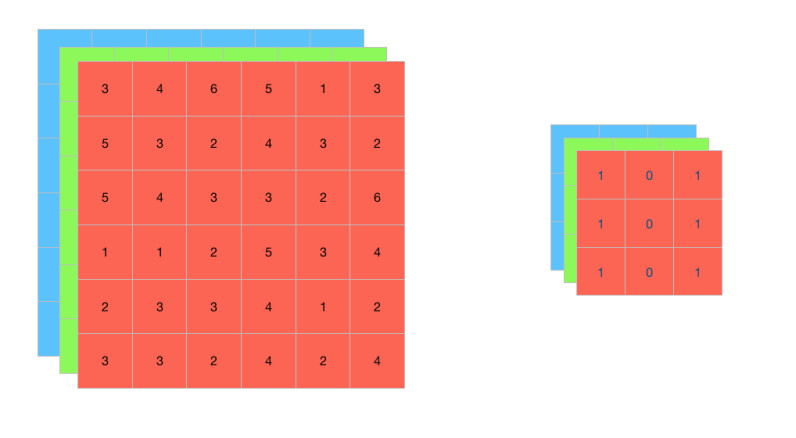Even though the convolutional layer has 3 kernels we end up with an output image of two dimensions, for example if we have an input image of size 6x6x3 and 3 kernels, each one of size 3x3x1, at the end these kernels sums its values, as a result we obtain an output image of size 4x4: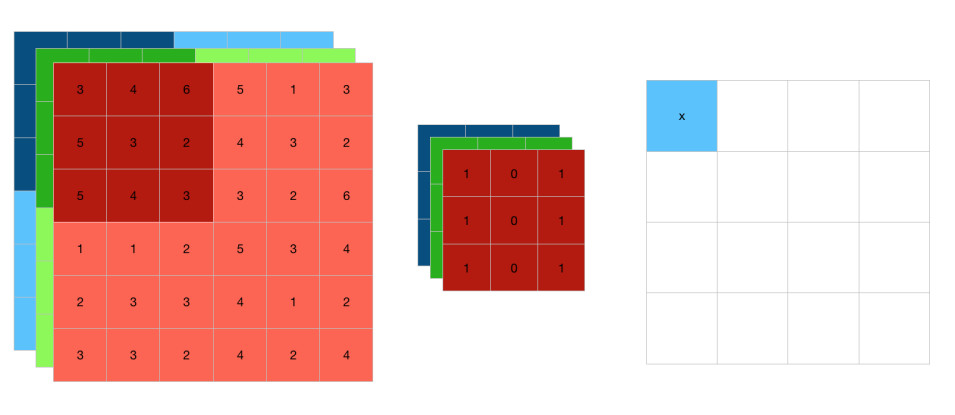In a convolutional layer we have several kernels to extract different features from the image, however, we usually don’t count the total number of kernels, for example if we say that a convolutional layer has 32 kernels we are counting the 3 kernels for each color channel as one, we could say that in total this convolutional layer has 96 kernels.

Pooling layer

In this layer we also use a kernel that passes over the image but this time the kernel picks the biggest value of the pixels, if we pass a kernel of size 2x2 and a stride of 2 over an image of size 4x4: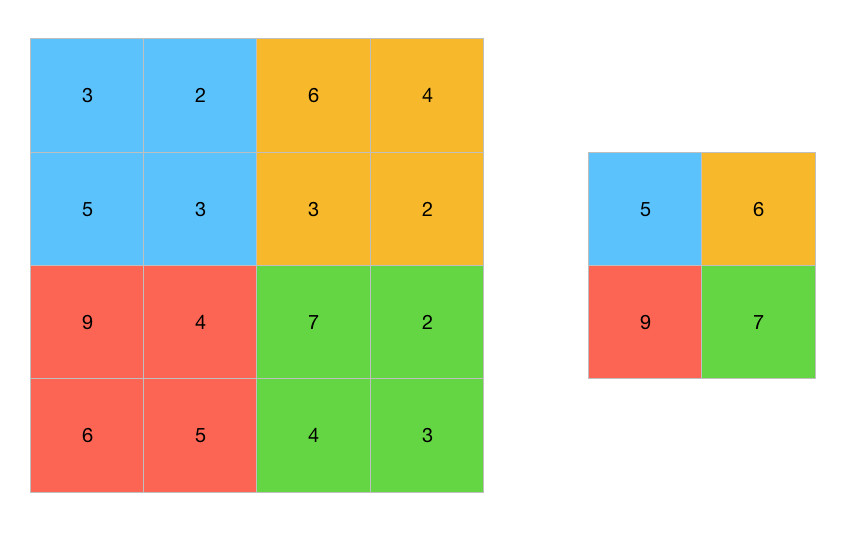We end up with an output image of size 2x2, the kernel picked the biggest value of each color section to form a new output image, this kind of pooling is called Max Pooling, we can also use a pooling called Average Pooling that picks the average of the pixels instead of the biggest value.

Pooling is a way of comprising an image, we usually use this layer after the convolutional layer.

Convolutional neural network

A convolutional neural network looks like: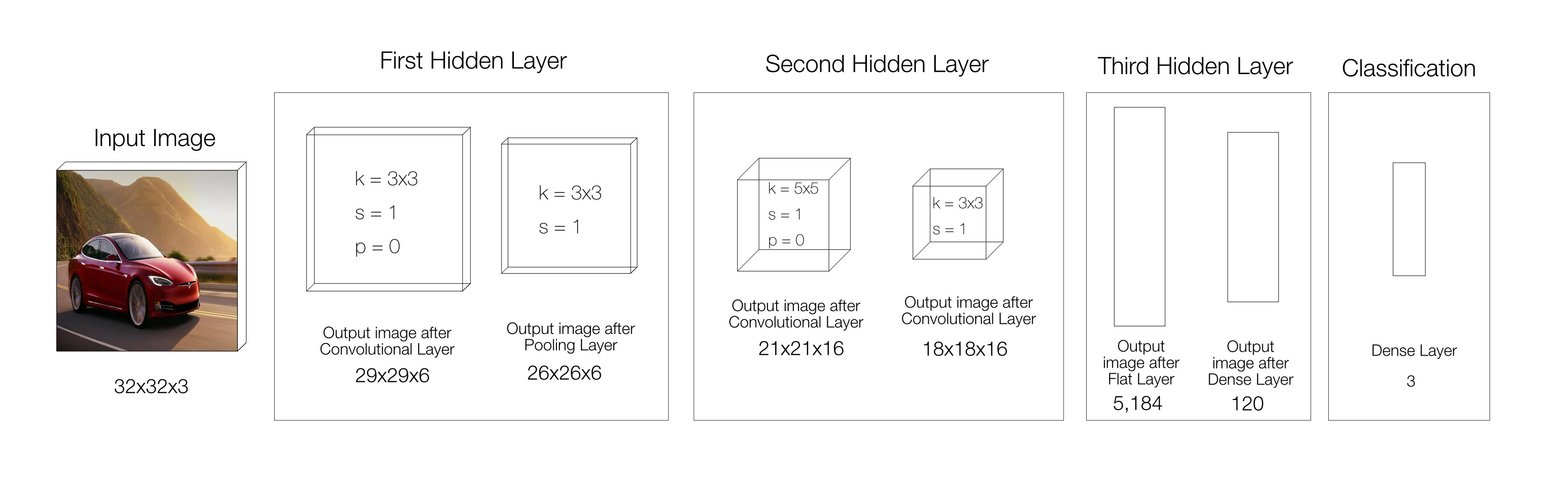We usually count the convolutional layer and the pooling layer as one, in this example we have a convolutional neural network with 3 hidden layers, 1 input layer and 1 output layer.

Input layer

In the input layer we have a color image of size 32x32x3.

First hidden layer

In the first hidden layer we have a convolutional layer with 6 kernels of size 3x3, a stride of 1 and no padding, the output image of this layer has a size of 29x29x6, then we have a pooling layer with one kernel of size 3x3 and a stride of 1, the output image has a size of 26x26x6.

Second hidden layer

In the second hidden layer we have one more convolutional layer, this time with 16 kernels of size 5x5 a stride of 1 and no padding, the output image of this layer has a size of 21x21x16, then we have a second pooling layer as well with one kernel of size 3x3 and a stride of 1, the output image has a size of 18x18x16.

Third hidden layer

Before performing the classification process, first we have to convert the output image of 3 dimensions to a flat layer of 1 dimension, we can multiple the dimensions 18 x 18 x 16 to obtain a flat layer of depth 5,184, then we can add a normal dense layer with 120 neurons.

Output layer

At the end we have a dense layer with 3 neurons, due to the fact we have 3 classes.

Keras

We can use Keras to build a convolutional neural network:

import keras
from keras.models import Sequential, Model
from keras.layers import Dense, Flatten, Activation
from keras.layers import Conv2D, MaxPooling2D

model = Sequential()

#First layer
activation='relu', strides=1, input_shape=(32, 32, 3))

#Second layer
activation='relu', strides=1))

#Third layer